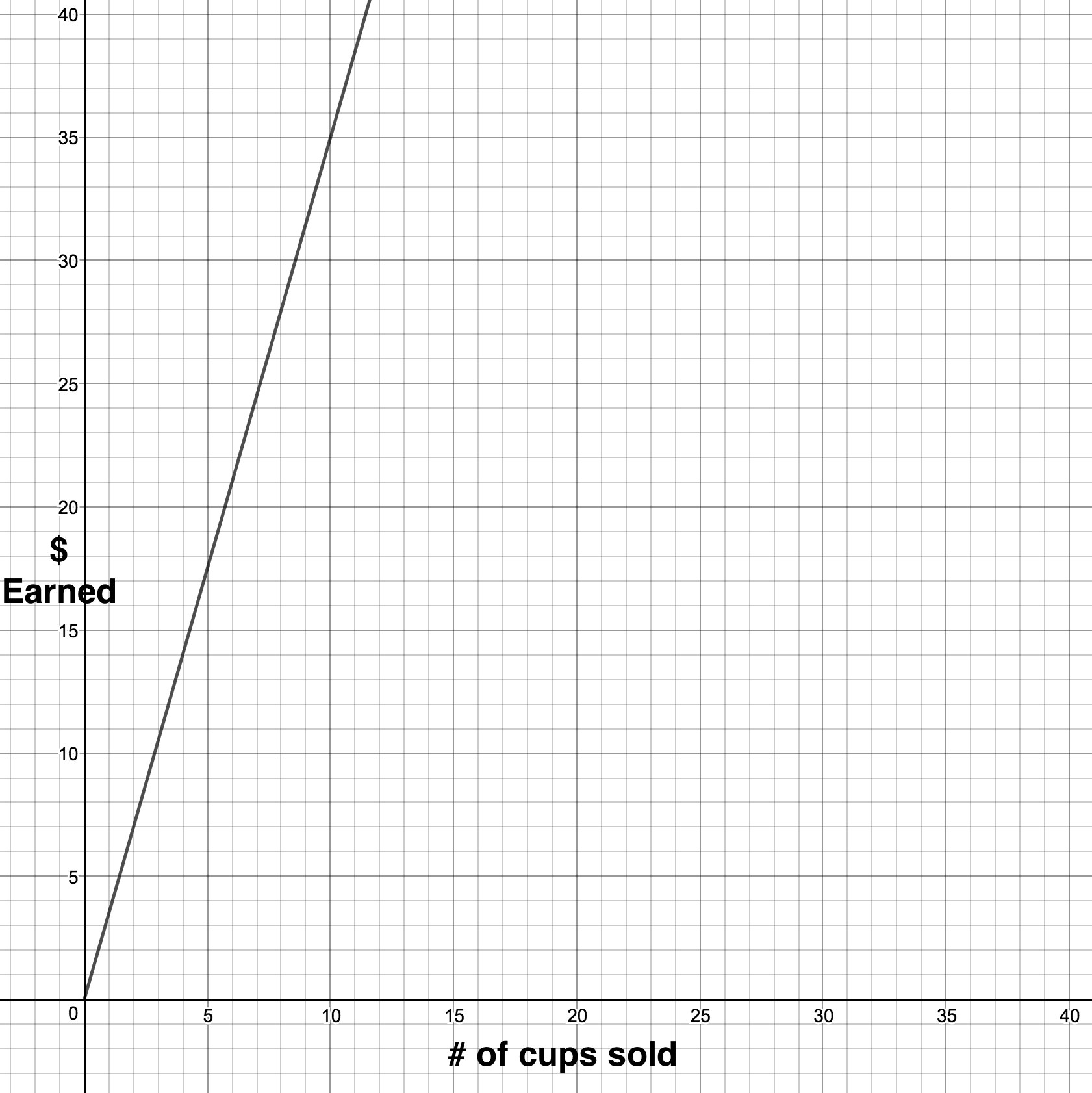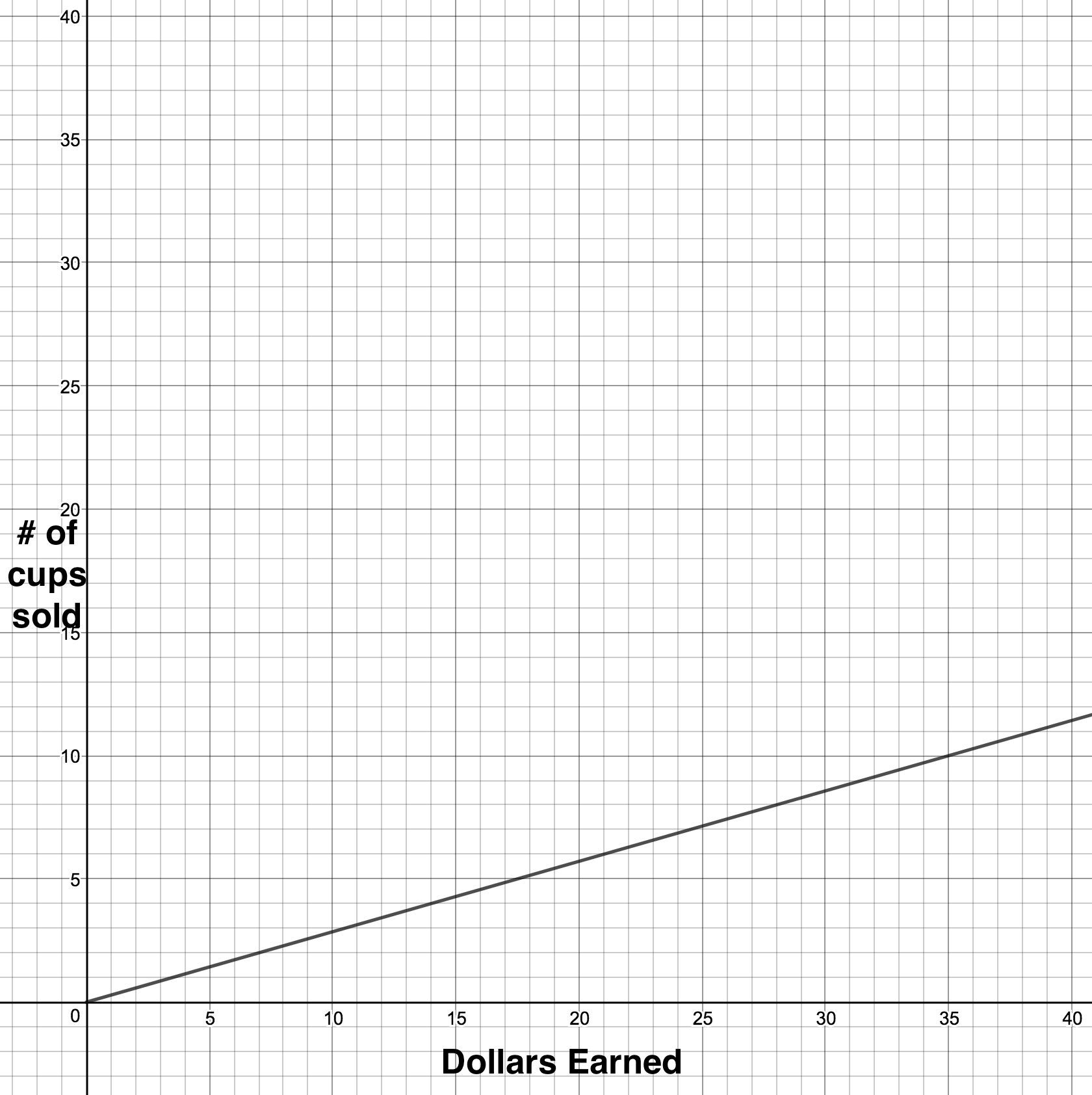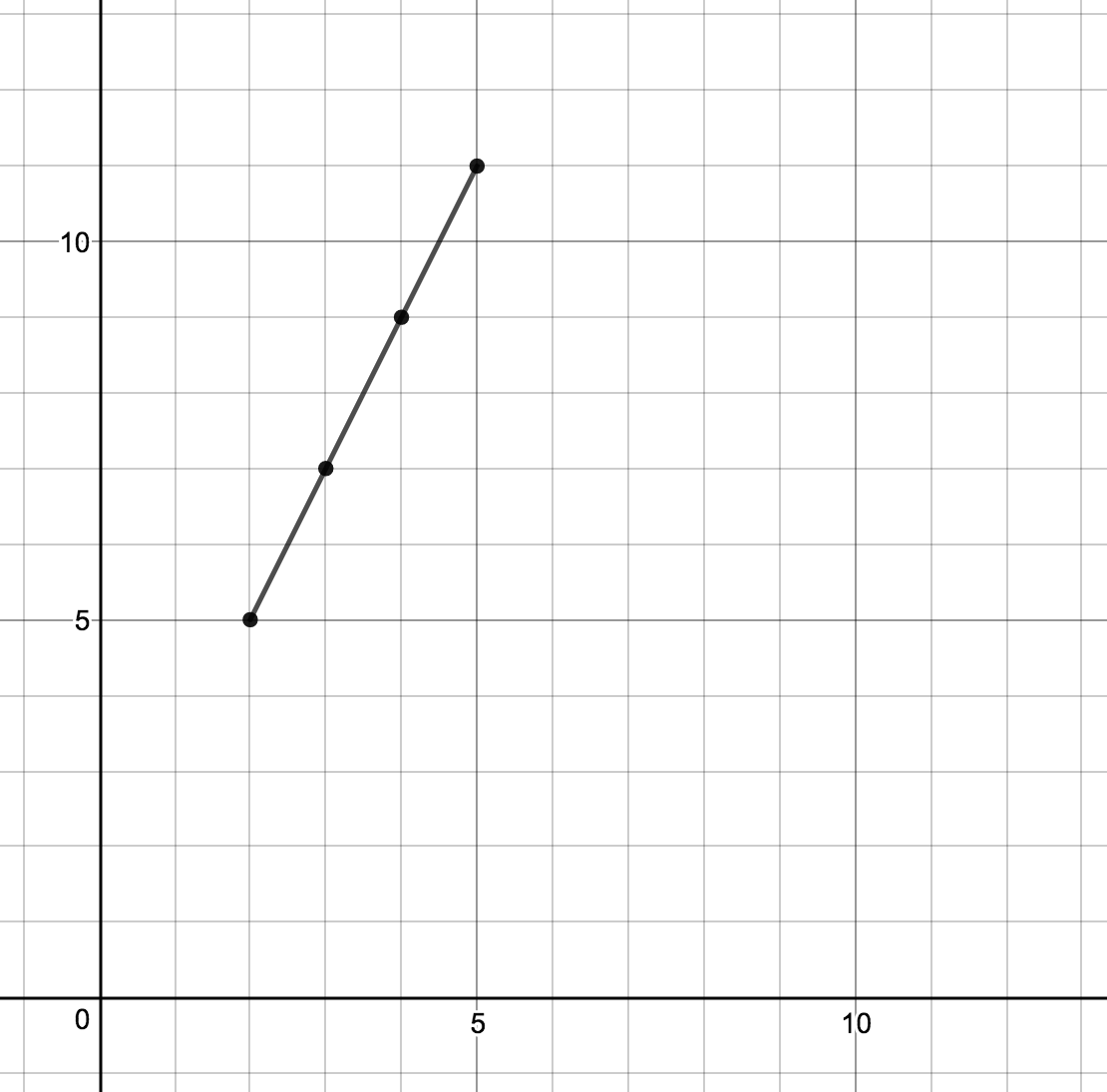# Linear Equations, Inequalities and Systems

## Objective

Identify inverse functions graphically and from a table of values in contextual and non-contextual situations.

## Common Core Standards

### Core Standards

?

• F.BF.B.4.A — Solve an equation of the form f(x) = c for a simple function f that has an inverse and write an expression for the inverse. For example, f(x) =2 x3 or f(x) = (x+1)/(x—1) for x ? 1.

• F.IF.A.1 — Understand that a function from one set (called the domain) to another set (called the range) assigns to each element of the domain exactly one element of the range. If f is a function and x is an element of its domain, then f(x) denotes the output of f corresponding to the input x. The graph of f is the graph of the equation y = f(x).

• F.IF.A.2 — Use function notation, evaluate functions for inputs in their domains, and interpret statements that use function notation in terms of a context.

• F.IF.B.5 — Relate the domain of a function to its graph and, where applicable, to the quantitative relationship it describes. For example, if the function h(n) gives the number of person-hours it takes to assemble n engines in a factory, then the positive integers would be an appropriate domain for the function. Modeling is best interpreted not as a collection of isolated topics but in relation to other standards. Making mathematical models is a Standard for Mathematical Practice, and specific modeling standards appear throughout the high school standards indicated by a star symbol (★). The star symbol sometimes appears on the heading for a group of standards; in that case, it should be understood to apply to all standards in that group.

?

• 8.F.A.1

• 8.F.B.4

## Criteria for Success

?

1. Describe an inverse function in a graph as a function that is reflected over the line ${y=x}$ and whose domain and range are also switched.
2. Compare the independent and dependent variables in a function and its inverse.
3. Use correct notation for inverse functions, ${f^{-1}(x)}$, and graph inverse functions.
4. Given a linear equation with a domain restriction, describe how the domain and range are affected in an inverse linear function.

## Anchor Problems

?

### Problem 1

Andrea is hosting a lemonade stand. She has graphed two functions that describe how much money she will make and how many cups of lemonade she will sell.How are the graphs and the functions they describe different from one another? Related to one another?

### Problem 2

Below is the function ${f(x) = 2x +1}$ where ${2\leq x< 5}$.Graph the inverse of the function, ${f^{-1}(x)}$, on the coordinate plane above.

## Problem Set

?

The following resources include problems and activities aligned to the objective of the lesson that can be used to create your own problem set.

• Include problems that use the context from the previous lesson. Give students the graph and ask them to find the inverse and describe what the inverse represents.
• Include problems where the two functions represented graphically are not inverse of one another. Ask students to describe how they know the two functions are not inverse of one another using the line of symmetry ${y=x}$ and the relationship between the coordinate points.

${(-2,-4)}$
${(1,-2.5)}$
${(2,-2)}$